Starts With A Bang

# Ask Ethan: How Dense Is A Black Hole?

#### It’s much more complex a question than dividing its mass by the volume of the event horizon. If you want to get a meaningful answer, you have to go deep.

If you took any massive object in the Universe and compressed it into a small enough volume, you could transform it into a black hole. Mass curves the fabric of space, and if you collect enough mass in a small enough region of space, that curvature will be so severe that nothing, not even light, can escape from it. The boundary of that inescapable regions is known as an event horizon, and the more massive a black hole is, the larger its event horizon will be. But what does that imply for the density of black holes? That’s what Patreon supporter Chad Marler wants to know, asking:

I have read that stellar-mass black holes are enormously dense, if you consider the volume of the black hole to be that space which is delineated by the event horizon, but that super-massive black holes are actually much less dense than even our own oceans. I understand that a black hole represents the greatest amount of entropy that can be squeezed into [any] region of space expressed… [so what happens to the density and entropy of two black holes when they merge]?

It’s a deep but fascinating question, and if we explore the answer, we can learn an awful lot about black holes, both inside and out.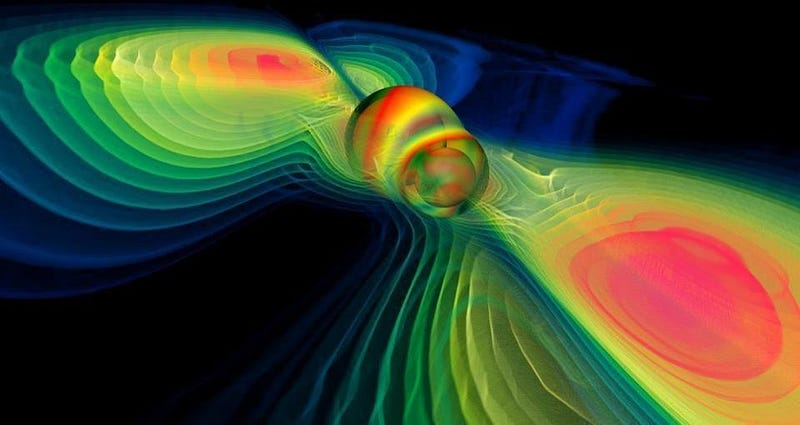Computer simulations enable us to predict which gravitational wave signals should arise from merging black holes. The question of what happens to the information encoded on the surfaces of the event horizons, though, is still a fascinating mystery. (WERNER BENGER, CC BY-SA 4.0)

Entropy and density are two very different things, and they’re both counterintuitive when it comes to black holes. Entropy, for a very long time, posed a big problem for physicists when they discussed black holes. Regardless of what you make a black hole out of — stars, atoms, normal matter, antimatter, charged or neutral or even exotic particles — only three properties matter for a black hole. Under the rules of General Relativity, black holes can have mass, electric charge, and angular momentum.

Once you make a black hole, all the information (and hence, all the entropy) associated with the components of the black hole are completely irrelevant to the end-state of a black hole that we observe. Only, if this were the true case, all black holes would have an entropy of 0, and black holes would violate the second law of thermodynamics.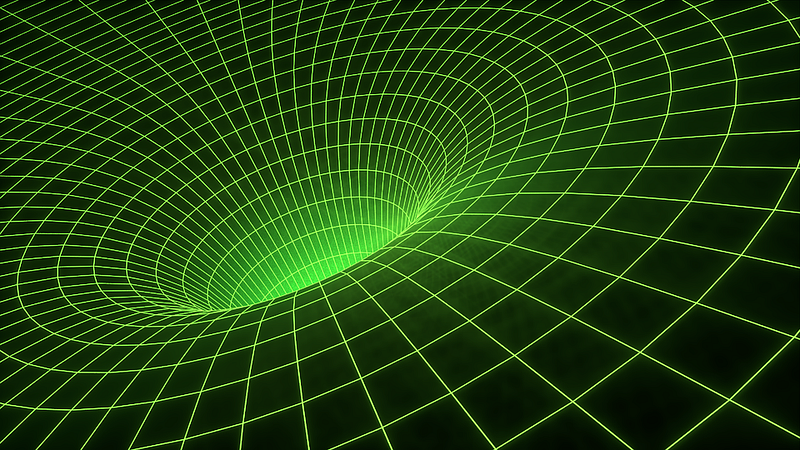An illustration of heavily curved spacetime, outside the event horizon of a black hole. As you get closer and closer to the mass’s location, space becomes more severely curved, creating a region where even light cannot escape: the event horizon. (PIXABAY USER JOHNSONMARTIN)

Similarly, we conventionally think of density as the amount of mass (or energy) contained within a given volume of space. For a black hole, the mass/energy content is easy to understand, since it’s the primary factor that determines the size of your black hole’s event horizon. Therefore, the minimum distance from the black hole where light (or any other) signals actually is defined by the radial distance from the black hole’s center to the edge of the event horizon.

This appears to give a natural scale for the volume of a black hole: the volume is determined by the amount of space enclosed by the surface area of the event horizon. A black hole’s density, consequently, can be obtained by dividing the mass/energy of the black hole by the volume of a sphere (or spheroid) that is found interior to the black hole’s event horizon. This is something that, at the very least, we know how to calculate.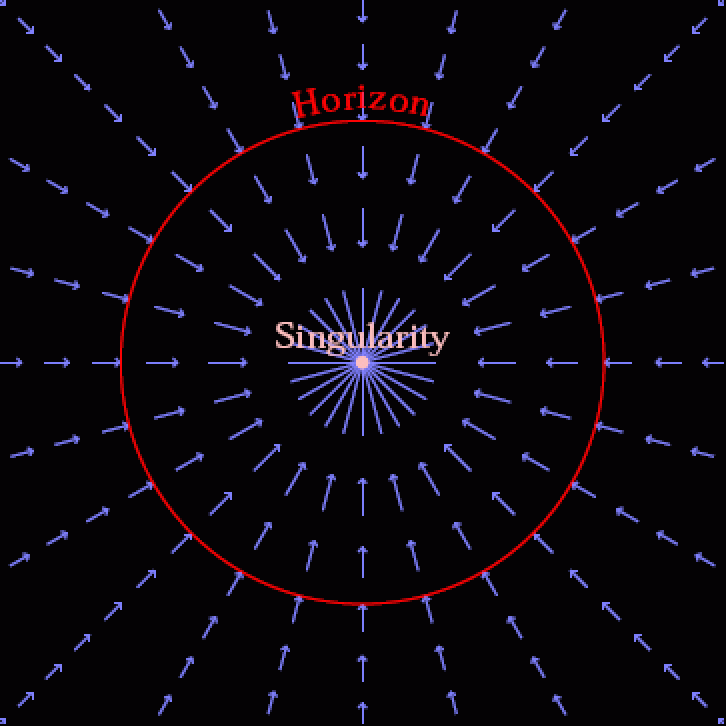Both inside and outside the event horizon, space flows like either a moving walkway or a waterfall, even through the event horizon itself. Upon crossing it, you are dragged inevitably to the central singularity. (ANDREW HAMILTON / JILA / UNIVERSITY OF COLORADO)

The question of entropy, in particular, poses a problem for physics as we understand it all on its own. If we can form a black hole (with zero entropy) out of matter (with non-zero entropy), then that means we destroy information, we lower the entropy of a closed system, and we violate the second law of thermodynamics. Any matter that falls into a black hole sees its entropy drop to zero; two neutron stars colliding to form a black hole sees the overall system’s energy plummet. Something is amiss.

But this was just a way of calculating a black hole’s entropy in General Relativity alone. If we add in the quantum rules that govern the particles and interactions in the Universe, we can immediately see that any particles that you’d either make a black hole from or add to the mass of a pre-existing black hole will have positive:

• temperatures,
• energies,
• and entropies.

Since entropy can never decrease, a black hole must have finite, non-zero, and positive entropy after all.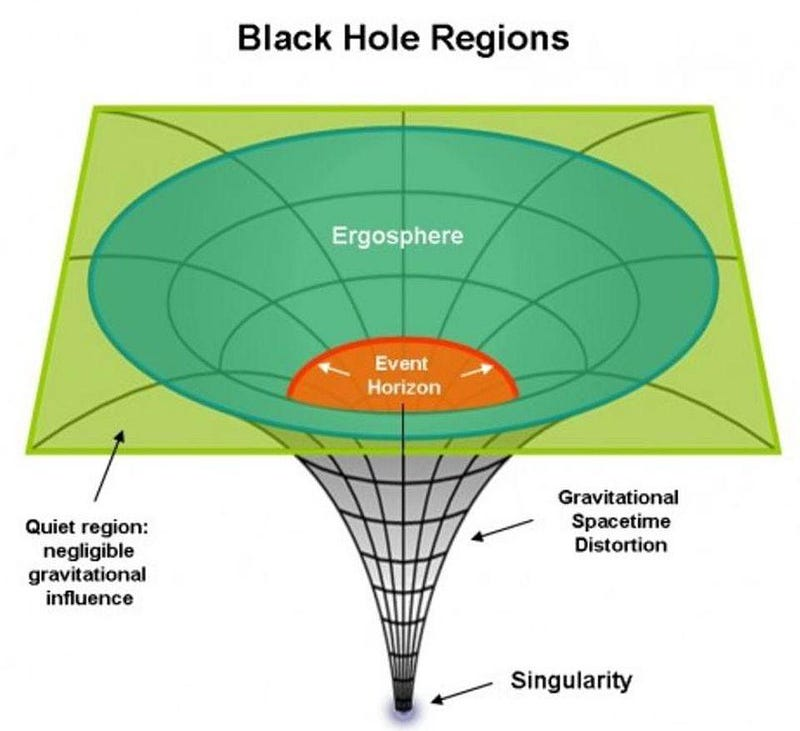Once you cross the threshold to form a black hole, everything inside the event horizon crunches down to a singularity that is, at most, one-dimensional. No 3D structures can survive intact. (ASK THE VAN / UIUC PHYSICS DEPARTMENT)

Whenever a quantum particle falls into (and passes across) a black hole’s event horizon, it will, at that moment, possess a number of particle properties inherent to it. These properties include angular momentum, charge, and mass, but they also include properties that black holes don’t appear to care about, such as polarization, baryon number, lepton number, and many others.

If the singularity at a black hole’s center doesn’t depend on those properties, there must be some other place capable of storing that information. John Wheeler was the first person to realize where it could be encoded: on the boundary of the event horizon itself. Instead of zero entropy, the entropy of a black hole would be defined by the number of quantum “bits” (or qubits) of information that could be encoded on the event horizon itself.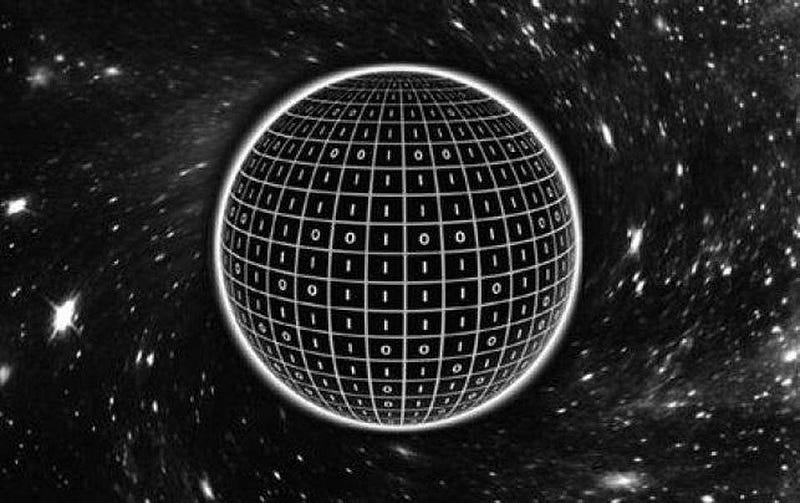Encoded on the outermost surface of the black hole, the event horizon, is its entropy. Each bit can be encoded on a surface area of the Planck length squared (~10^-66 m²); a black hole’s total entropy is given by the Bekenstein-Hawking formula. (T.B. BAKKER / DR. J.P. VAN DER SCHAAR, UNIVERSITEIT VAN AMSTERDAM)

Given that a black hole will have an event horizon with a surface area that’s proportional to the size of its radius squared (since mass and radius are directly proportional for black holes), and that the surface area required to encode one bit is the Planck length squared (~10^-66 m²), the entropy of even a small, low-mass black hole is enormous. If you were to double the mass of a black hole, you’d double its radius, which means its surface area would now be four times its previous value.

If you compare the lowest-mass black holes we know of — which are somewhere in the ballpark of 3-to-5 solar masses — to the highest-mass ones (of tens of billions of solar masses), you’ll find enormous differences in entropy. Entropy, remember, is all about the number of possible quantum states a system can be configured in. For a 1 solar-mass black hole whose information is encoded on its surface, the entropy is approximately 10⁷⁸ k_b (where k_b is Boltzmann’s constant), with more massive black holes having that number increase by a factor of (M_BH/M_Sun)². For the black hole at the center of the Milky Way, the entropy is around 10⁹¹ k_b, while for the supermassive one at the center of M87 — the first one imaged by the Event Horizon Telescope — the entropy is a little more than 10⁹⁷ k_b. The entropy of a black hole is, indeed, the maximum possible amount of entropy that can exist within a given particular region of space.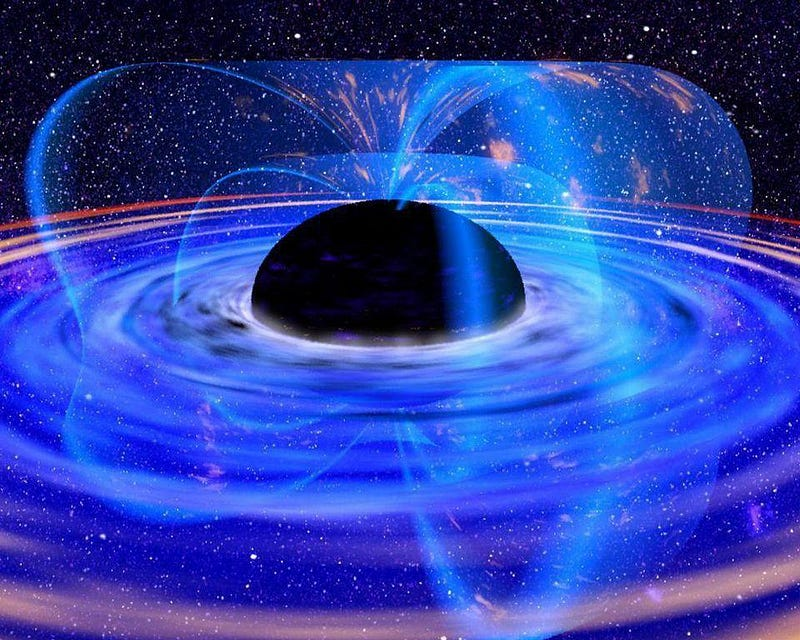The event horizon of a black hole is a spheroidal region from which nothing, not even light, can escape. Although conventional radiation originates outside the event horizon, it is unclear how the encoded entropy behaves in a merger scenario. (NASA; DANA BERRY, SKYWORKS DIGITAL, INC)

As you can see, the more massive your black hole is, the more entropy (proportional to mass squared) it possesses.

But then we come to density, and all our expectations break down. For a black hole of a given mass, its radius will be directly proportional to the mass, but the volume is proportional to the radius cubed. A black hole the mass of Earth would be just a little under 1 cm in radius; a black hole the mass of the Sun would be about 3 km in radius; the black hole at the center of the Milky Way is approximately 10⁷ km in radius (about 10 times the radius of the Sun); the black hole at the center of M87 weighs in at a little bit over 10¹⁰ km in radius, or about half a light-day.

This means, if we were to calculate density by dividing the mass of a black hole by the volume it occupies, we’d find that the density of a black hole (in units of kg/m³) with the mass of:

• the Earth is 2 × 10³⁰ kg/m³,
• the Sun is 2 × 10¹⁹ kg/m³,
• the Milky Way’s central black hole is 1 × 10⁶ kg/m³, and
• M87’s central black hole is ~1 kg/m³,

where that last value is about the same as density of air on Earth’s surface.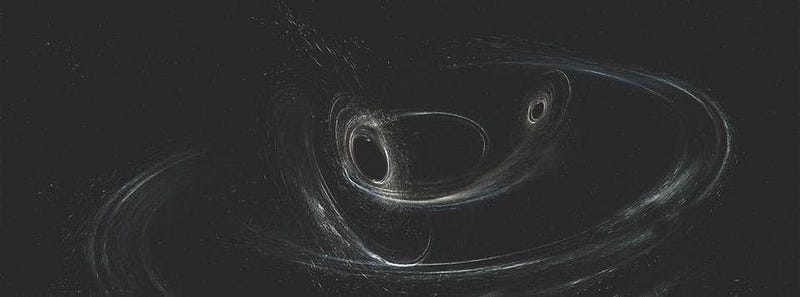For real black holes in our Universe, we can observe the radiation emitted by their surrounding matter, and the gravitational waves produced by the inspiral, merger, and ringdown. Where the entropy/information goes is not yet determined. (LIGO/CALTECH/MIT/SONOMA STATE (AURORE SIMONNET))

Are we to believe, then, that if we take two black holes of some roughly equal masses, and allow them to inspiral and merge together, that

1. The entropy of the final black hole will be four times the entropy of each initial black hole,
2. While the density of the final black hole will be one-fourth the density of each of the initial black holes?

The answers, perhaps surprisingly, are “Yes” and “No,” respectively.

For entropy, it is indeed true that merging a black hole (of mass M and entropy S) with another equal mass black hole (of mass M and entropy S) will give you a new black hole with double the mass (2M) but four times the entropy (4S), exactly as predicted by the Bekenstein-Hawking equation. If we calculate how the entropy of the Universe has evolved over time, it’s increased by approximately 15 orders of magnitude (a quadrillion) from the Big Bang until today. Almost all of that extra entropy is in the form of black holes; even the Milky Way’s central black hole has about 1,000 times the entropy of the entire Universe as it was immediately following the Big Bang.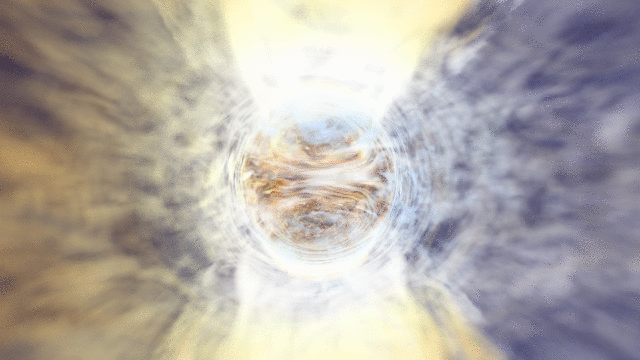From outside a black hole, all the infalling matter will emit light and always is visible, while nothing from behind the event horizon can get out. But that does not mean a black hole’s density is uniform inside the event horizon. (ANDREW HAMILTON, JILA, UNIVERSITY OF COLORADO)

For density, however, it’s neither fair nor correct to take the mass of a black hole and divide it by the volume inside the event horizon. Black holes are not solid, uniform-density objects, and the laws of physics inside a black hole are expected to be no different than the laws of physics outside. The only difference is the strength of the conditions and the curvature of space, which means that any particles that fall in past the boundary of the event horizon will continue falling until they can fall no longer.

From outside a black hole, all you can see is the boundary of the event horizon, but the most extreme conditions found in the Universe occur in the interiors of black holes. To the best of our knowledge, falling into a black hole — across the event horizon — means that you’ll inevitably head towards the central singularity in a black hole, something that’s an inescapable fate. If your black hole is non-rotating, the singularity is nothing but a mere point. If all the mass is compressed into a single, zero-dimensional point, then when you ask about density, you are asking “what happens when you divide a finite value (mass) by zero?”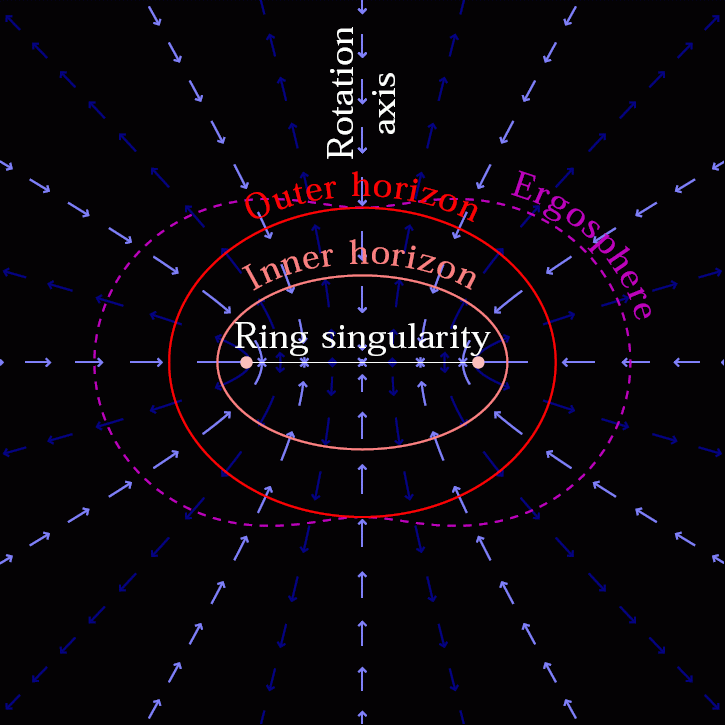Spacetime flows continuously both outside and inside the (outer) event horizon for a rotating black hole, similar to the non-rotating case. The central singularity is a ring, rather than a point, while simulations break down at the inner horizon. (ANDREW HAMILTON / JILA / UNIVERSITY OF COLORADO)

If you need a reminder, dividing by zero is mathematically bad; you get an undefined answer. Thankfully, perhaps, non-rotating black holes aren’t what we have in our physical Universe. Our realistic black holes rotate, and that means that the interior structure is much more complicated. Instead of a perfectly spherical event horizon, we get a spheroidal one that’s elongated along its plane of rotation. Instead of a point-like (zero-dimensional) singularity, we get a ring-like (one-dimensional) one, which is proportional to the angular momentum (and the angular momentum-to-mass) ratio.

But perhaps most interestingly, when we examine the physics of a rotating black hole, we find that there isn’t one solution for an event horizon, but two: an inner and an outer horizon. The outer horizon is what we physically call the “event horizon” and what we observe with telescopes like the Event Horizon Telescope. But the inner horizon, if we understand our physics correctly, is actually inaccessible. Any object that falls into a black hole will see the laws of physics break down as it approaches that region of space.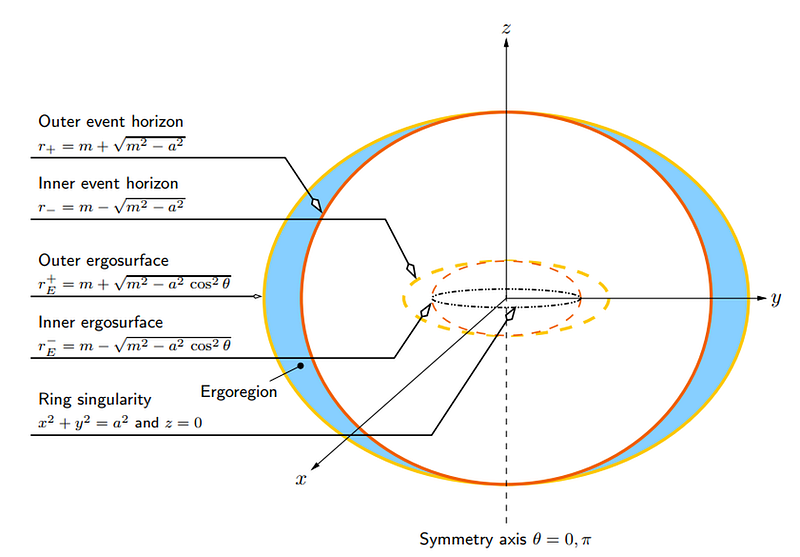The exact solution for a black hole with both mass and angular momentum was found by Roy Kerr in 1963. Instead of a single event horizon with a point-like singularity, we get inner and outer event horizons, ergospheres, plus a ring-like singularity. (MATT VISSER, ARXIV:0706.0622)

All the mass, charge, and angular momentum of a black hole is contained in a region even an infalling observer cannot access, but the size of that region varies dependent on how large the angular momentum is, up to some maximum value (as a percentage of mass). The black holes we’ve observed are largely consistent with having angular momenta at or near that maximum value, so even though the “volume” we cannot access inside is smaller than the event horizon, it still increases precipitously (as mass squared) as we look to more and more massive black holes. Even the size of the ring singularity increases in direct proportion to mass, so long as the mass-to-angular momentum ratio remains constant.

But there is no contradiction here, just some counterintuitive behavior. It teaches us that we probably can’t split a black hole in two without getting a whole bunch of extra entropy out. It teaches us that using a quantity like density for a black hole means we have to be careful, and are irresponsible if we just divide its mass by the event horizon’s volume. And it teaches us, if we bother to calculate it, that the spatial curvature at the event horizon is enormous for low-mass black holes, but barely discernible for high-mass black holes. A non-rotating black hole has an infinite density, but a rotating one will have its mass spread out across a ring-like shape, with the rotational rate and the total mass determining the black hole’s linear density.

Unfortunately for us, there’s no way we know of to test this experimentally or observationally. We might be able to calculate — to help us visualize — what we theoretically expect to happen inside of a black hole, but there’s no way to get the observational evidence.Travel the Universe with astrophysicist Ethan Siegel. Subscribers will get the newsletter every Saturday. All aboard!

The closest we’ll be able to come is to look to gravitational wave detectors like LIGO, Virgo and KAGRA, and to measure the ringdowns (i.e., the physics in the immediate aftermath) of two merging black holes. It can help confirm certain details that will either validate or refute our current best picture of black hole interiors. So far, everything lines up exactly as Einstein predicted, and exactly as theorists expected.

There’s still a lot to learn about what happens when two black holes merge, even for quantities like density and entropy, which we think we understand. With more and better data pouring in — and improved data on the near-term horizon — it’s almost time to start putting our assumptions to the ultimate experimental tests!

Send in your Ask Ethan questions to startswithabang at gmail dot com!

Ethan Siegel is the author of Beyond the Galaxy and Treknology. You can pre-order his third book, currently in development: the Encyclopaedia Cosmologica.

Related
Biology plays an important role in emotional reactions, but neuroscientist Kristen A. Lindquist posits that our culture is just as influential.
7 min
with
Yes, you CAN be a “math person” — as long as you follow these learning techniques.
4 min
with
Ancient organic chemistry.
“Only a narcissist would want to become president.” This is the psychology of an authoritarian unpacked.
11 min
with
Combining years of neurological research and mindfulness techniques, Dr. Heather Berlin helps us better understand how the body’s most complex organ can easily be misled into negative thinking - and how we can stop that from happening.
with

Up Next
"On the spectrum from worry to action, parents can choose to act," a new report states.